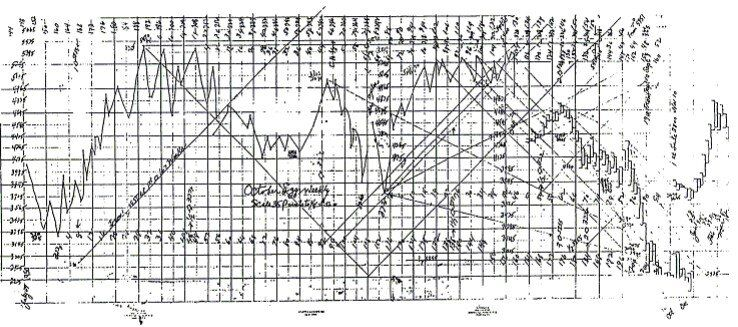# How to Draw Gann Angles Correctly in Easy Way

Gann angles play an essential part to read the market trend. Angles were one of the main trading tools of WD Gann.

He used to trade with Gann angles to see top and bottoms in the market, to find support and resistance, and for the trend.

The first and always most important Gann angle is 45 degree.

The 45-degree angle divides the space and time into two equal parts. It moves one point per day, week or month (depends on the time frame you are drawing).

The stock price above 45 degrees Gann angle shows the trend is strong. And the price below the 45-degree angle means a weak trend.

The other vital angles after 45 degrees 1*1 are 2*1,3*1, 4*1, 8*1, 16*1 angles.To draw Gann angles correctly, you need the proper square chart. But in today’s time, the right square charts are next to impossible.

WD Gann draws the chart through his hand, which were proper square charts. So if you can draw the chart through your hand then only you can correctly draw Gann angles.

You can use Gannalyst or Optuma Market analyst Gann software's to draw Gann angles. But still, such angles will not the same as Gann use to draw.

There are many other ways to use Gann angle numbers. Gann had told the calculation based way also to use Gann angles.

For such, you need the Gann angle number. And then add such Gann angle number to the significant tops or bottoms to locate essential levels. You will also find many times the market will make the high and lows exact on such calculated levels.

Frankly, there are many many ways to use and apply Gann angles in trading. The list is long.

I prefer to draw angles by considering ‘TIME’. Such a way is compelling and allows me to draw angle without square charts. That angles also work for Intraday trading. And it’s easy.

I teach to draw that Gann angles in my ‘Master Course’ if you wish you can learn by joining my ‘Master Course’.